##### How to Use MIN() Function in PostgreSQL

PostgreSQL offers a built-in MIN() function that retrieves a set's minimum value. It takes a column’s name as an argument and returns the least value of the targeted set. The specified column’s type can be a string, number, or any other comparable data type.

Let’s learn the working of the MIN() function through Practical examples.

How to Use MIN() Function in Postgres?

The below snippet will show you the basic syntax of the MIN() function:

`MIN(exp);`

Here, exp represents an expression. Let's implement it practically to gain a deeper understanding of the MIN() function.

Example #1: How to Use the MIN() Function on Table’s Data?

Our database already has a table named bike_details. Here are the table details:

`SELECT * FROM bike_details;`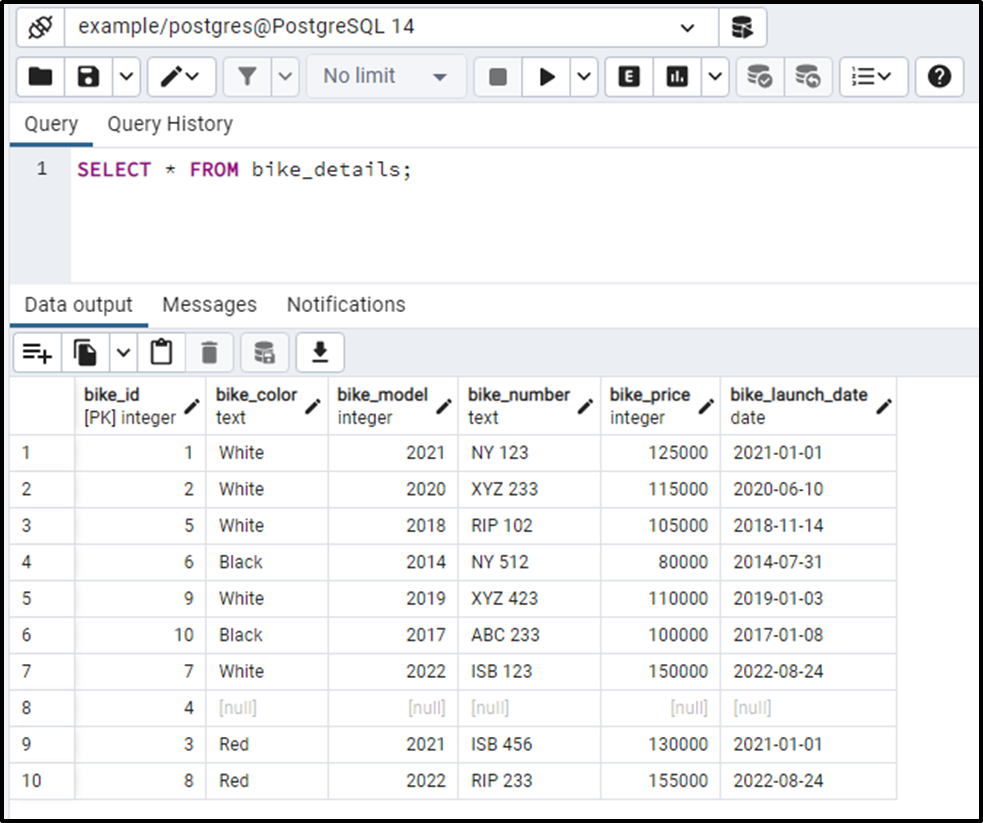Let’s find the bike with the minimum price. To do that, we will use the Postgres MIN() Function:

```SELECT MIN(bike_price)
FROM bike_details;```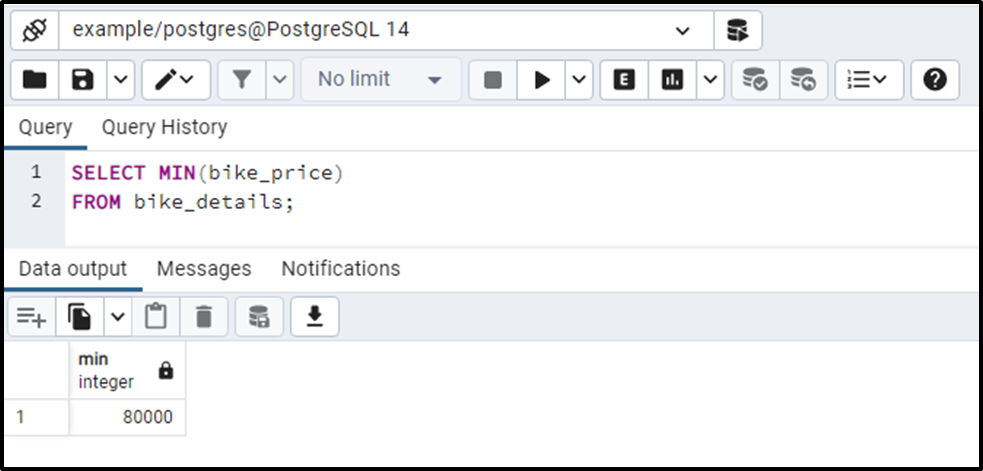The output shows that the bike_details table's most cost-effective bike costs 80000.

Example #2: How to Use MIN() Function With a Subquery?

Suppose we want to fetch all the details about the most cost-effective bike. To do so, we will use the MIN() function with a subquery as follows:

```SELECT * FROM bike_details
WHERE bike_price = (
SELECT MIN(bike_price)
FROM bike_details
);```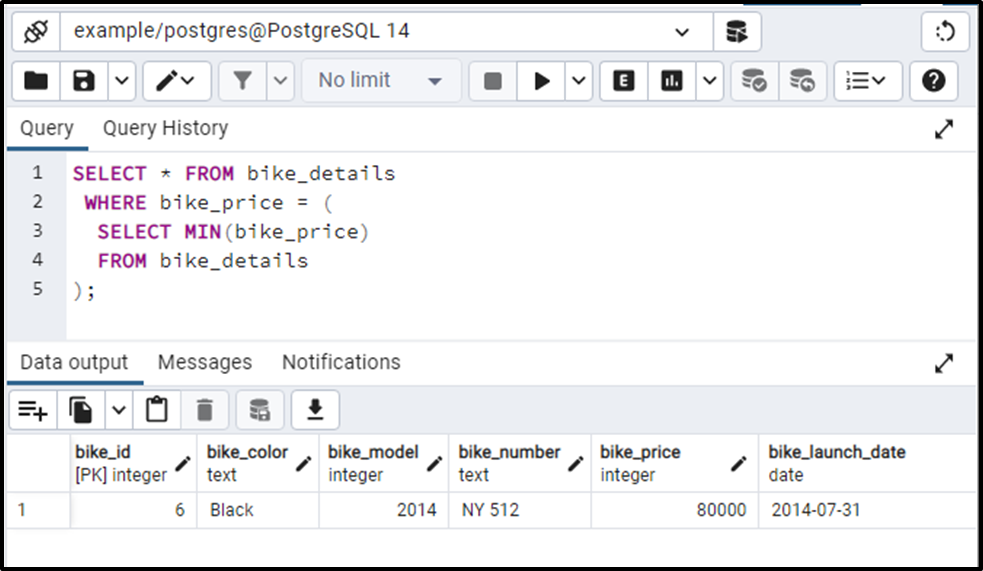This is how the MIN() function works with the subquery in Postgres.

Example #3: How to Use the MIN() Function With GROUP BY Clause?

The following command will return the bike with the least price from each group. Let’s utilize the GROUP BY clause as follows:

```SELECT
bike_model,
MIN(bike_price)
FROM bike_details
GROUP BY bike_model;```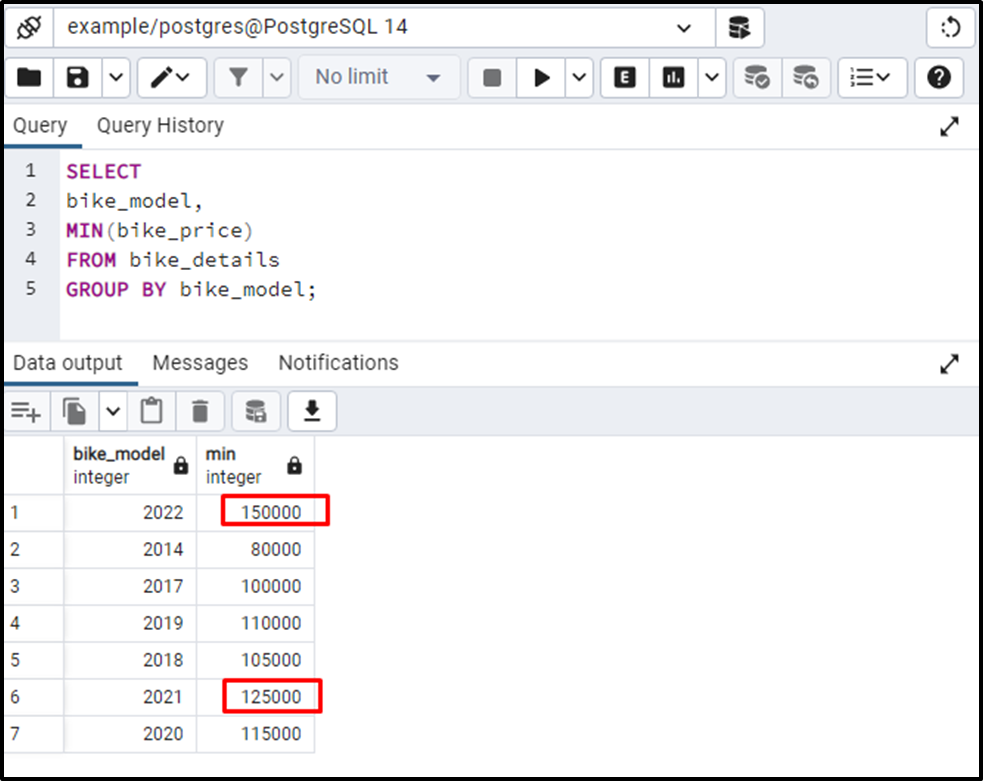From the output, you can observe that this time the MIN() function returned the minimum value for each group.

Example #4: How to Use the MIN() Function With HAVING Clause?

We can specify a special condition with the MIN() function using the HAVING clause:

``` SELECT
bike_model,
MIN (bike_price)
FROM
bike_details
GROUP BY
bike_model
HAVING MIN(bike_price) >80000;```

In this example, we utilized the MIN() function along with the GROUP BY clause to find the bike with the least price from each group. Next, we utilized the HAVING clause specifying that the bike price must be greater than 80000. Here is the final outcome of the above query: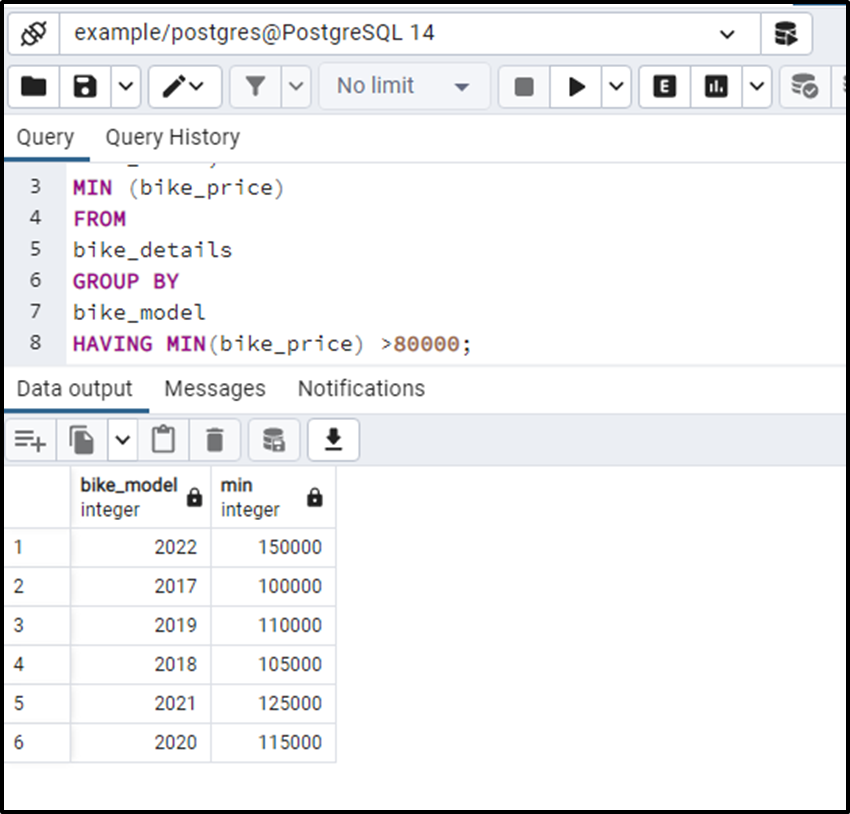The output shows that the MIN() function retrieves the bikes having the least price in their respective group. However, it skipped the bikes having a price less than or equal to 80000.

Example #5: How to Get the MINIMUM Value From Multiple Columns?

Use the LEAST() function to get the minimum/least value from more than one column. We have modified the bike_details table by inserting a new column in it. The below-given command will show the modified table:

`SELECT * FROM bike_details;`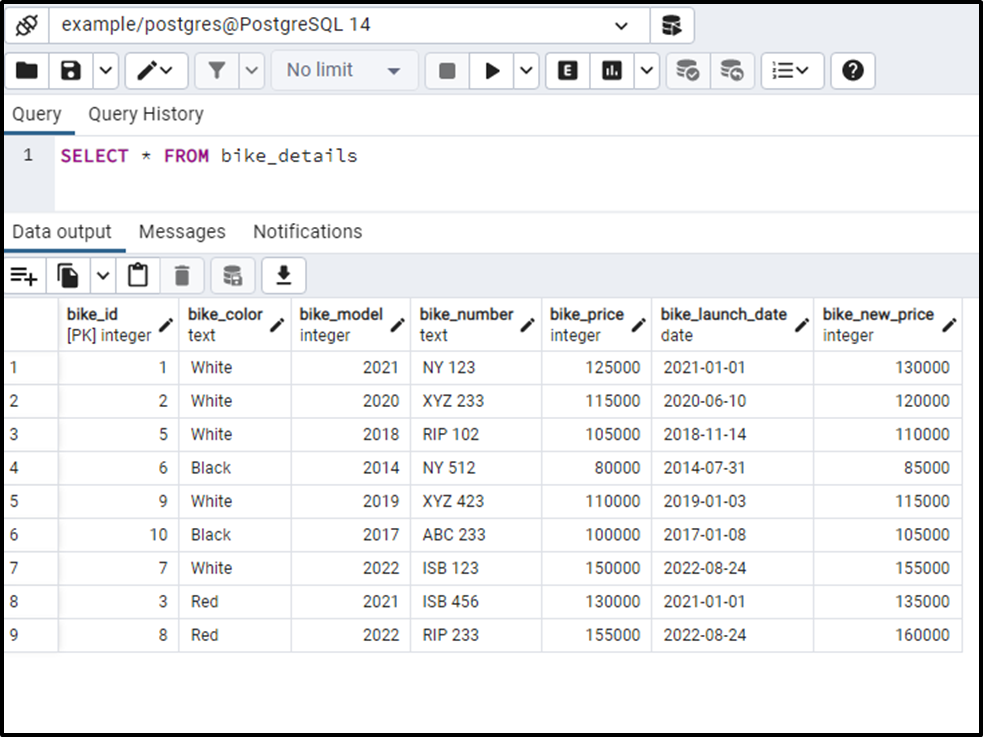Let’s utilize the LEAST() function to fetch the least value from the bike_price and bike_new_price columns:

```SELECT
bike_model,
LEAST(bike_price, bike_new_price) AS least_price
FROM
bike_details;```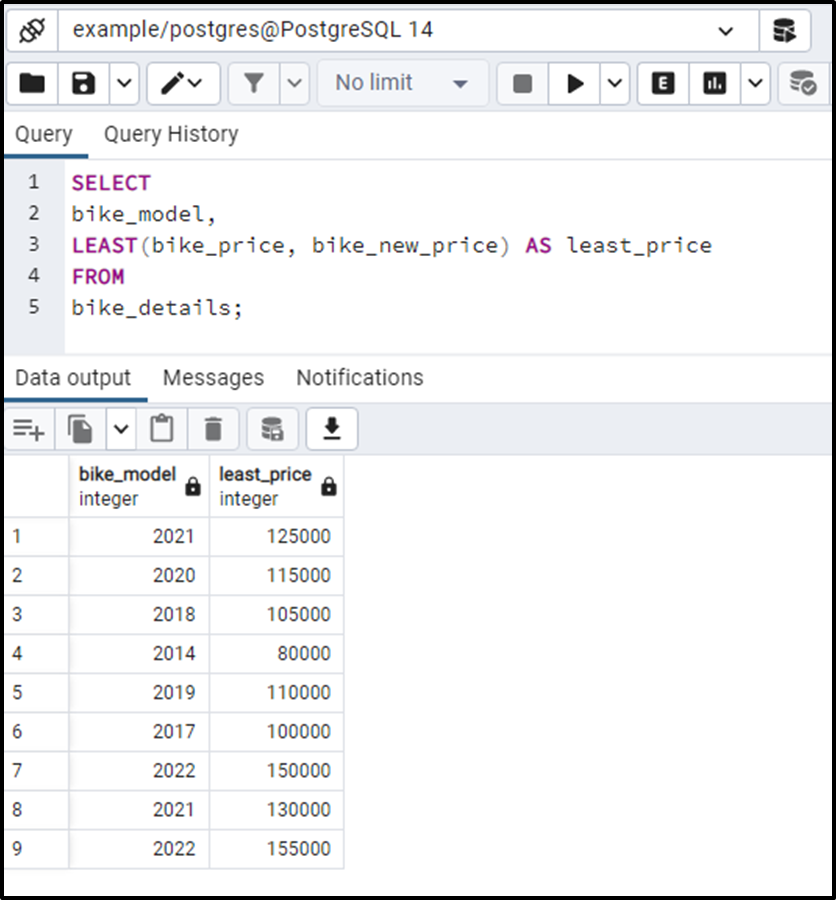In this way, you can find the least value from multiple columns using the LEAST() function.

Conclusion

PostgreSQL provides an in-built function named MIN() that retrieves a set's minimum value. Use the LEAST() function to get the minimum/least value from more than one column. In this post, we have learned how to use the MIN() function with the HAVING clause, GROUP BY clause, and subquery. This write-up considered different examples to demonstrate the working of the MIN() function in PostgreSQL.